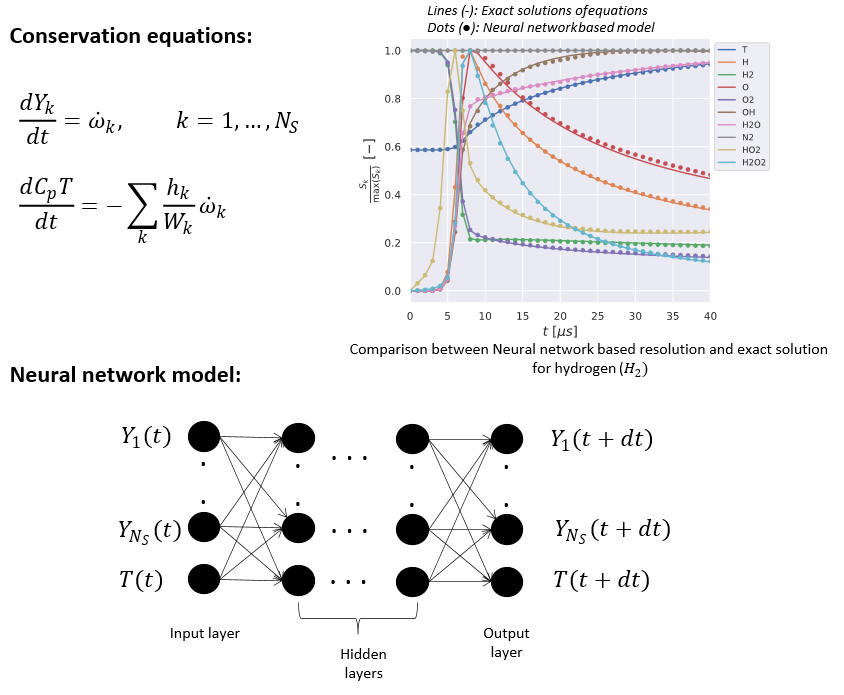Numerical simulations are now widely employed in the industrial world to help design systems and predict complex phenomena. Reactive flow simulation, for example, is important for numerous applications, such as vehicle and aircraft propulsion and processes in the chemicals industry.

Reactive flows involve mixtures of chemical constituents and compounds that react with each other resulting in, depending on the nature of these reactions, an evolution of species over periods of time that can vary widely. Describing the temporal evolution of these species within the flow requires the use of advanced numerical methods - i.e., costly calculation times - and solving these equations accounts for a significant part of the total simulation time for a system.

The use of learning methods to speed up chemical kinetics calculations is an approach that has recently gained in popularity. When calculating an industrial system, the idea is to replace traditional resolution algorithms with an equivalent model, derived from a learning process, that can be evaluated more quickly. This model is generated by optimizing a set of parameters from a database of exact, previously simulated solutions.

To do this, researchers at IFPEN used neural networks, due to their capacity to reproduce the evolution of chemical species. The research focused on two areas.

• The first compared different neural network structures to predict the evolutions of chemical species. Standard networks were compared to so-called “recurrent” neural networks, making it possible to establish predictions taking into account a set of past values, rather than a single value.

• The second focused on physicochemical constraints, in particular the need to respect the conservation of mass for each element present in the mixture, something that is not guaranteed with neural networks traditionally used.

The methodology deployed was evaluated for the homogeneous combustion of hydrogen. In this situation, since the mass fractions do not vary in space, numerical resolution is limited to time-related differential equations, as illustrated in the figure.

• Concerning the evolutions over time of the chemical species (figure ), very good agreement was observed between the exact solutions and the solutions obtained with neural networks . Increased accuracy was also observed for recurrent networks.

• A method was devised to construct neural networks that guarantee the conservation of mass of chemical elements .Illustration of the neural network resolution approach for the combustion of H2

This research illustrates the capacity of neural networks to replace chemical kinetics resolution algorithms. Future work on the method will focus on the following aspects:

• Deployment for cases of practical interest, through the definition of adapted learning databases.

• Evaluation of the calculation time savings compared to traditional methods.

Publications:

  M. Guirat, T. Faney, C. Mehl, Modeling of chemical evolution equations using Long Short Term Memory Neural Networks, publication submitted.

 C. Mehl, T. Faney, patent under examination.

Scientific contact: cedric.mehl@ifpen.fr

>> ISSUE 45 OF SCIENCE@IFPEN